### Problem Set 3 Arithmetic Progression Class 10th Mathematics Part 1 MHB Solution

Problem Set 3

1. The sequence - 10, - 6, - 2, 2, . . . Choose the correct alternative answer for each…
2. First four terms of an A.P. are ....., whose first term is - 2 and common difference…
3. What is the sum of the first 30 natural numbers ? Choose the correct alternative…
4. For an given A.P. t7 = 4, d = - 4 then a = . . . Choose the correct alternative answer…
5. For an given A.P. a = 3.5, d = 0, n = 101, then tn = . . . Choose the correct…
6. In an A.P. first two terms are - 3, 4 then 21st term is . . . Choose the correct…
7. If for any A.P. d = 5 then t18 - t13 = ... Choose the correct alternative answer for…
8. Sum of first five multiples of 3 is. . . Choose the correct alternative answer for…
9. 15, 10, 5, . . . In this A.P. sum of first 10 terms is . . . Choose the correct…
10. In an A.P. 1st term is 1 and the last term is 20. The sum of all terms is = 399 then n…
11. Find the fourth term from the end in an A.P. - 11, - 8, - 5, . . ., 49.…
12. In an A.P. the 10th term is 46, sum of the 5th and 7th term is 52. Find the A.P.…
13. The A.P. in which 4th term is - 15 and 9th term is - 30. Find the sum of the first 10…
14. Two A.P.’s are given 9, 7, 5, . . . and 24, 21, 18, . . . . If nth term of both the…
15. If sum of 3rd and 8th terms of an A.P. is 7 and sum of 7th and 14th terms is - 3 then…
16. In an A.P. the first term is - 5 and last term is 45. If sum of all numbers in the A.P.…
17. Sum of 1 to n natural numbers is 36, then find the value of n.
18. Divide 207 in three parts, such that all parts are in A.P. and product of two smaller…
19. There are 37 terms in an A.P., the sum of three terms placed exactly at the middle is…
20. If first term of an A.P. is a, second term is b and last term is c, then show that sum…
21. If the sum of first p terms of an A.P. is equal to the sum of first q terms then show…
22. If m times the mth term of an A.P. is equal to n times nth term then show that the (m…
23. ₹ 1000 is invested at 10 percent simple interest. Check at the end of every year if…

###### Problem Set 3
Question 1.

Choose the correct alternative answer for each of the following sub questions.

The sequence – 10, – 6, – 2, 2, . . .
A. is an A.P., Reason d = – 16

B. is an A.P., Reason d = 4

C. is an A.P., Reason d = – 4

D. is not an A.P.

First term a = – 10

Second term t1 = – 6

Third term t2 = – 2

Fourth term t3 = 2

Common difference d = t1 – a = – 6 – ( – 10) = – 6 + 10 = 4

Common difference d = t2 – t1 = – 2 – ( – 6) = – 2 + 6 = 4

Common difference d = t3 – t2 = 2 – ( – 2) = 2 + 2 = 4

Since, the common difference is same

∴ The given sequence is A.P. with common difference d = 4

Question 2.

Choose the correct alternative answer for each of the following sub questions.

First four terms of an A.P. are ....., whose first term is – 2 and common difference is – 2.
A. – 2, 0, 2, 4

B. – 2, 4, – 8, 16

C. – 2, – 4, – 6, – 8

D. – 2, – 4, – 8, – 16

Given first term t1 = – 2

Common difference d = – 2

By using formula tn + 1 = tn + d

t2 = t1 + d = – 2 + ( – 2) = – 2 – 2 = – 4

t3 = t2 + d = – 4 + ( – 2) = – 4 – 2 = – 6

t4 = t3 + d = – 6 + ( – 2) = – 6 – 2 = – 8

Hence, the A.P. is – 2, – 4, – 6, – 8

Question 3.

Choose the correct alternative answer for each of the following sub questions.

What is the sum of the first 30 natural numbers ?
A. 464

B. 465

C. 462

D. 461

List of first 30 natural number is

1, 2, 3,……..,30

First term a = 1

Second term t1 = 2

Third term t2 = 3

Common difference d = t3 – t2 = 3 – 2 = 1

number of terms n = 30

Thus, By using sum of nth term of an A.P. we will find it’s sum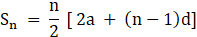Where, n = no. of terms

a = first term

d = common difference

Sn = sum of n terms

We need to find S30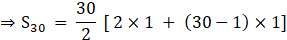⇒ S30 = 15 [ 2 + 29]

⇒ S30 = 15 × 31

⇒ S30 = 465

Question 4.

Choose the correct alternative answer for each of the following sub questions.

For an given A.P. t7 = 4, d = – 4 then a = . . .
A. 6

B. 7

C. 20

D. 28

Now, By using nth term of an A.P. formula

tn = a + (n – 1)d

where n = no. of terms

a = first term

d = common difference

tn = nth terms

⇒ t7 = a + (7 – 1) × ( – 4)

⇒ 4 = a + 6 × ( – 4)

⇒ 4 = a – 24

⇒ a = 24 + 4 = 28

Thus, the correct answer is (D)

Question 5.

Choose the correct alternative answer for each of the following sub questions.

For an given A.P. a = 3.5, d = 0, n = 101, then tn = . . .
A. 0

B. 3.5

C. 103.5

D. 104.5

Given: a = 3.5, d = 0, n = 101

Now, By using nth term of an A.P. formula

tn = a + (n – 1)d

where n = no. of terms

a = first term

d = common difference

tn = nth terms

Substituting all given value in the formulae we get,

⇒ tn = 3.5 + (101 – 1) × 0

⇒ tn = 3.5

Question 6.

Choose the correct alternative answer for each of the following sub questions.

In an A.P. first two terms are – 3, 4 then 21st term is . . .
A. – 143

B. 143

C. 137

D. 17

Given: first term a = – 3

Second term t1 = 4

Common difference d = t1 – a = 4 – ( – 3) = 4 + 3 = 7

We need to find t21 where n = 21

Now, By using nth term of an A.P. formula

tn = a + (n – 1)d

where n = no. of terms

a = first term

d = common difference

tn = nth terms

Substituting all given value in the formulae we get,

⇒ t21 = – 3 + (21 – 1) × 7

⇒ t21 = – 3 + 20 × 7

⇒ t21 = – 3 + 140 = 137

Question 7.

Choose the correct alternative answer for each of the following sub questions.

If for any A.P. d = 5 then t18 – t13 = ...
A. 5

B. 20

C. 25

D. 30

Given d = 5

Now, By using nth term of an A.P. formula

tn = a + (n – 1)d

where n = no. of terms

a = first term

d = common difference

tn = nth terms

Thus, t18 – t13 = [a + (18 – 1) × 5] – [ a + (13 – 1) × 5]

⇒ t18 – t13 = [ 17 × 5] – [ 12 × 5]

⇒ t18 – t13 = 85 – 60 = 25

Question 8.

Choose the correct alternative answer for each of the following sub questions.

Sum of first five multiples of 3 is. . .
A. 45

B. 55

C. 15

D. 75

First five multiples of 3 are

3, 6, 9, 12, 15

First term a = 3

Second term t1 = 6

Third term t2 = 9

Common difference d = t2 – t1 = 9 – 6 = 3

Thus, By using sum of nth term of an A.P. we will find it’s sumWhere, n = no. of terms

a = first term

d = common difference

Sn = sum of n terms

We need to find S5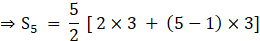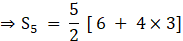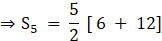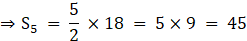Question 9.

Choose the correct alternative answer for each of the following sub questions.

15, 10, 5, . . . In this A.P. sum of first 10 terms is . . .
A. – 75

B. – 125

C. 75

D. 125

First term a = 15

Second term t1 = 10

Third term t2 = 5

Common difference d = t2 – t1 = 5 – 10 = – 5

No. of terms n = 10

Thus, By using sum of nth term of an A.P. we will find it’s sumWhere, n = no. of terms

a = first term

d = common difference

Sn = sum of n terms

We need to find S10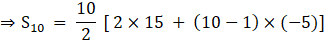⇒S10 = 5 [ 30 + 9 × ( – 5)]

⇒S10 = 5 [ 30 – 45]

⇒S10 = 5 × ( – 15) = – 75

Question 10.

Choose the correct alternative answer for each of the following sub questions.

In an A.P. 1st term is 1 and the last term is 20. The sum of all terms is = 399 then n = . . .
A. 42

B. 38

C. 21

D. 19

Given, first term = 1

Last term = 20

Sum of n terms, Sn = 399

We need to find no. of terms n

Using Sum of n terms of an A.P. formula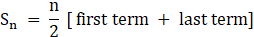where n = no. of terms

Sn = sum of n terms

Now, on substituting given value in formula we get,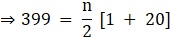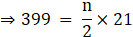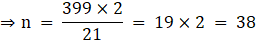Question 11.

Find the fourth term from the end in an A.P. – 11, – 8, – 5, . . ., 49.

First term from end a = 49

tn = – 11

tn – 1 = – 8

Common difference d = tn – tn – 1 = – 11 – ( – 8) = – 11 + 8 = – 3

Now, By using nth term of an A.P. formula

tn = a + (n – 1)d

where n = no. of terms

a = first term

d = common difference

tn = nth terms

no. of terms n = 4

⇒ t4 = 49 + (4 – 1) × ( – 3)

⇒ t4 = 49 + 3 × ( – 3)

⇒ t4 = 49 – 9 = 40

Question 12.

In an A.P. the 10th term is 46, sum of the 5th and 7th term is 52. Find the A.P.

Given: t10 = 46

t5 + t7 = 52

Now, By using nth term of an A.P. formula

tn = a + (n – 1)d

where n = no. of terms

a = first term

d = common difference

tn = nth terms

Hence, by given condition we get,

⇒ t10 = 46

⇒ a + (10 – 1)d = 46

⇒ a + 9d = 46 ……(1)

⇒ t5 + t7 = 52

⇒ [a + (5 – 1)d] + [a + (7 – 1)d] = 52

⇒ [a + 4d] + [a + 6d] = 52

⇒ 2a + 10d = 52 ……(2)

Multiply eq. (2) by 2 we get,

⇒ 2a + 18d = 92 ……(3)

Subtract eq. (2) by eq. (3)

⇒ [2a + 18d] – [ 2a + 10d] = 92 – 52

⇒ 8d = 40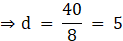Substitute “d” in (1)

⇒ a + 9 × 5 = 46

⇒ a + 45 = 46

⇒ a = t1 = 46 – 45 = 1

we know that, tn + 1 = tn + d

⇒ t2 = t1 + d = 1 + 5 = 6

⇒ t3 = t2 + d = 6 + 5 = 11

Hence, an A.P. is 1, 6, 11, . . .

Question 13.

The A.P. in which 4th term is – 15 and 9th term is – 30. Find the sum of the first 10 numbers.

t4 = – 15 and t9 = – 30

Now, By using nth term of an A.P. formula

tn = a + (n – 1)d

where n = no. of terms

a = first term

d = common difference

tn = nth terms

Hence, by given condition we get,

t4 = – 15

⇒ a + (4 – 1)d = – 15

⇒ a + 3d = – 15 …..(1)

t9 = – 30

⇒ a + (9 – 1)d = – 30

⇒ a + 8d = – 30 …..(2)

Subtracting eq. (1) from eq. (2)

⇒ [a + 8d] – [a + 3d] = – 30 – ( – 15)

⇒ 5d = – 30 + 15 = – 15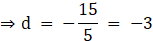Substituting, “d” in eq. (1)

⇒ a + 3 × ( – 3) = – 15

⇒ a + – 9 = – 15

⇒ a = – 15 + 9 = – 6

Thus, By using sum of nth term of an A.P. we will find it’s sumWhere, n = no. of terms

a = first term

d = common difference

Sn = sum of n terms

We need to find S10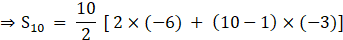⇒S10 = 5 [ – 12 + 9 × ( – 3)]

⇒S10 = 5 [ – 12 – 27]

⇒S10 = 5 × ( – 39) = – 195

Question 14.

Two A.P.’s are given 9, 7, 5, . . . and 24, 21, 18, . . . . If nth term of both the progressions are equal then find the value of n and nth term.

Given A.P. is 9, 7, 5….

Whose first tern a = 9

Second term t1 = 7

Third term t3 = 5

Common difference d = t3 – t2 = 5 – 7 = – 2

Another A.P. is 24, 21, 18, . . .

Whose first tern a = 24

Second term t1 = 21

Third term t3 = 18

Common difference d = t3 – t2 = 18 – 21 = – 3

We have been given, nth term of both the A.P. is same

thus, by using nth term of an A.P. formula

tn = a + (n – 1)d

where n = no. of terms

a = first term

d = common difference

tn = nth terms

Hence, by given condition we get,

⇒ 9 + (n – 1) × ( – 2) = 24 + (n – 1) × ( – 3)

⇒ 9 – 2n + 2 = 24 – 3n + 3

⇒ 11 – 2n = 27 – 3n

⇒ 3n – 2n = 27 – 11

⇒ n = 16

Thus, value of nth term where a = 9, d = – 2, n = 16 is

⇒ tn = 9 + (16 – 1) × ( – 2)

⇒ tn = 9 – 15 × 2

⇒ tn = 9 – 30 = – 21

Question 15.

If sum of 3rd and 8th terms of an A.P. is 7 and sum of 7th and 14th terms is – 3 then find the 10th term.

Now, By using nth term of an A.P. formula

tn = a + (n – 1)d

where n = no. of terms

a = first term

d = common difference

tn = nth terms

Hence, by given condition we get,

t3 + t8 = 7

⇒ [a + (3 – 1)d] + [a + (8 – 1)d] = 7

⇒ [a + 2d] + [a + 7d] = 7

⇒ 2a + 9d = 7 …..(1)

t7 + t14 = – 3

⇒ [a + (7 – 1)d] + [a + (14 – 1)d] = – 3

⇒ [a + 6d] + [ a + 13d] = – 3

⇒ 2a + 19d = – 3 …..(2)

Subtracting eq. (1) from eq. (2)

⇒ [2a + 19d] – [2a + 9d] = – 3 – 7

⇒ 10d = – 10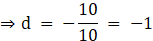Substituting, “d” in eq. (1)

⇒ 2a + 9 × ( – 1) = 7

⇒ 2a – 9 = 7

⇒ 2a = 7 + 9 = 16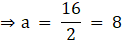Now, we can find value of t10

Thus, t10 = 8 + (10 – 1)× ( – 1)

⇒ t10 = 8 – 9 = – 1

Question 16.

In an A.P. the first term is – 5 and last term is 45. If sum of all numbers in the A.P. is 120, then how many terms are there? What is the common difference?

Given, first term a = – 5

Last term tn = 45

Sum of n terms Sn = 120

To find no of terms “n”

Using Sum of n terms of an A.P. formulawhere n = no. of terms

Sn = sum of n terms

Now, on substituting given value in formula we get,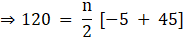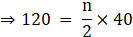⇒ 120 = 20 n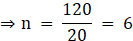To find the common difference ‘d’

We use nth term of an A.P. formula

tn = a + (n – 1)d

where n = no. of terms

a = first term

d = common difference

tn = nth terms

Thus, on substituting all values we get,

⇒ t6 = – 5 + (6 – 1)d

⇒ 45 = – 5 + 5d

⇒ 5d = 45 + 5 = 50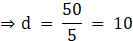Thus, common difference is 10

Question 17.

Sum of 1 to n natural numbers is 36, then find the value of n.

List of n natural number is

1, 2, 3, ……n

First term a = 1

Second term t1 = 2

Third term t3 = 3

Thus, common difference d = t3 – t2 = 3 – 2 = 1

Given Sn = 36

Thus, By using sum of nth term of an A.P. we will find it’s sumWhere, n = no. of terms

a = first term

d = common difference

Sn = sum of n terms

We need to find no. of terms n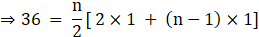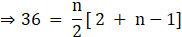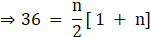⇒ n(1 + n) = 36 × 2 = 72

⇒ n2 + n – 72 = 0

⇒ n2 + 9n – 8n – 72 = 0

⇒ n(n + 9) – 8(n + 9) = 0

⇒ (n – 8)(n + 9) = 0

⇒ n – 8 = 0 or n + 9 = 0

⇒ n = 8 or n = – 9

Since, number of terms n can’t be negative

∴ n = 8

Question 18.

Divide 207 in three parts, such that all parts are in A.P. and product of two smaller parts will be 4623.

Let 3 parts of 207 be a – d, a, a + d such that,

⇒ (a – d) + a + (a + d) = 207

⇒ 3a = 207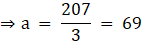Since, product of two smaller terms is 4623

⇒ (a – d) × a = 4623

⇒ (69 – d) × 69 = 4623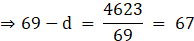⇒ d = 69 – 67 = 2

Thus, a – d = 69 – 2 = 67

a = 69

a + d = 69 + 2 = 71

Thus, the A.P so formed is 67, 69, 71

Question 19.

There are 37 terms in an A.P., the sum of three terms placed exactly at the middle is 225 and the sum of last three terms is 429. Write the A.P.

Let first term = a

Common difference = d

Since, A.P. consist of 37 terms, therefor the middle most term is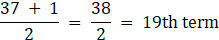Thus, three middle most term are t18 = 18th term, t19 = 19th term,

t20 = 20th term

We use nth term of an A.P. formula

tn = a + (n – 1)d

where n = no. of terms

a = first term

d = common difference

tn = nth terms

Thus, on substituting all values we get,

Given, t18 + t19 + t20 = 225

⇒ [a + (18 – 1)d] + [a + (19 – 1)d] + [a + (20 – 1)d] = 225

⇒ [a + 17d] + [a + 18d] + [a + 19d] = 225

⇒ 3a + 54d = 225

Dividing by 3

⇒ a + 18d = 75 …….(1)

Given, sum of last three term is 429

⇒ t35 + t36 + t37 = 429

⇒ [a + (35 – 1)d] + [a + (36 – 1)d] + [a + (37 – 1)d] = 429

⇒ [a + 34d] + [a + 35d] + [a + 36d] = 429

⇒ 3a + 105d = 429

Dividing by 3

⇒ a + 35d = 143 …….(2)

Subtracting eq. (1) from eq. (2) we get,

⇒ [a + 35d] – [a + 18d] = 143 – 75

⇒ 17d = 68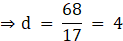Substituting value of ‘d’ in eq. (1) we get,

⇒ a + 18 × 4 = 75

⇒ a + 72 = 75

⇒ a = 75 – 72 = 3

⇒ a = t1 = 3

We know that, tn + 1 = tn + d

t2 = t1 + d = 3 + 4 = 7

t3 = t2 + d = 7 + 4 = 11

t4 = t3 + d = 11 + 4 = 15

t37 = 3 + (37 – 1) × 4

t37 = 3 + 36 × 4

t37 = 3 + 144 = 147

Thus, the A.P. is 3, 7, 11, . . . . ., 147

Question 20.

If first term of an A.P. is a, second term is b and last term is c, then show that sum of all terms is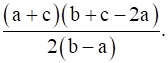Given first term = a

Second term = b

Last term = c

Common difference d = second term – first term = b – a

We will first find the number of terms

We use nth term of an A.P. formula

tn = a + (n – 1)d

where n = no. of terms

a = first term

d = common difference

tn = nth terms

Thus, on substituting all values we get,

⇒ c = a + (n – 1)(b – a)

⇒ c = a + (b – a)n + a – b

⇒ c = 2a – b + (b – a)n

⇒ (b – a)n = c + b – 2a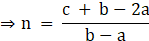Using Sum of n terms of an A.P. formulawhere n = no. of terms

Sn = sum of n terms

On substituting all the values we get,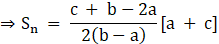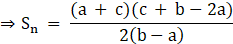Hence, proved

Question 21.

If the sum of first p terms of an A.P. is equal to the sum of first q terms then show that the sum of its first (p + q) terms is zero. (p ≠ q)

We know that, sum of nth term of an A.P. we will find it’s

sumWhere, n = no. of terms

a = first term

d = common difference

Sn = sum of n terms

Now, Sum of p terms is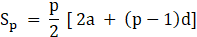And, Sum of q terms is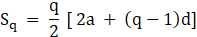Given: Sp = Sq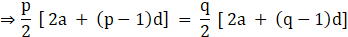Multiply by 2 on both sides, we get,

⇒p[ 2a + (p – 1)d] = q[ 2a + (q – 1)d]

⇒2ap + p(p – 1)d = 2aq + q(q – 1)d

⇒ 2ap – 2aq + p(p – 1)d – q(q – 1)d = 0

⇒ 2a(p – q) + d[p2 – p– q2 + q] = 0

⇒ 2a(p – q) + d[(p2– q2 ) – p + q] = 0

⇒ 2a(p – q) + d[(p– q )(p + q) – (p – q)] = 0

(since, (a – b)(a + b) = a2 – b2)

⇒ 2a(p – q) + d(p – q) [p + q – 1 ] = 0

⇒ (p – q)[2a + d (p + q – 1) ] = 0

Since, p ≠ q

∴ p – q ≠ 0

⇒ 2a + d (p + q – 1) = 0

Multiply both side by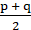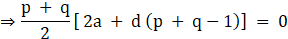⇒ Sp + q = 0

Hence proved

Question 22.

If m times the mth term of an A.P. is equal to n times nth term then show that the (m + n)th term of the A.P. is zero.

We use nth term of an A.P. formula

tn = a + (n – 1)d

where n = no. of terms

a = first term

d = common difference

tn = nth terms

Thus mth term = tm = a + (m – 1)d

Given: m × tm = n × tn

⇒ m × [ a + (m – 1)d] = n × [a + (n – 1)d]

⇒ am + m(m – 1)d = an + n(n – 1)d

⇒ am – an + m(m – 1)d – n(n – 1)d = 0

⇒ a(m – n) + d[m(m – 1) – n(n – 1)] = 0

⇒ a(m – n) + d[ m2 – m – n2 + n] = 0

⇒ a(m – n) + d[ (m2 – n2) – m + n] = 0

⇒ a(m – n) + d[ (m – n)(m + n) –(m – n)] = 0

(since, (a – b)(a + b) = a2 – b2)

⇒ a(m – n) + d(m – n)[(m + n) –1] = 0

⇒ (m – n) [a + d(m + n –1)] = 0

Since, m ≠ n

∴ m – n ≠ 0

⇒ a + d(m + n –1) = 0

⇒ tm + n = 0

Hence proved

Question 23.

₹ 1000 is invested at 10 percent simple interest. Check at the end of every year if the total interest amount is in A.P. If this is an A.P. then find interest amount after 20 years. For this complete the following activity.

Simple interest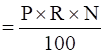Simple interest after 1 year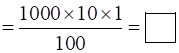Simple interest after 2 year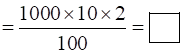Simple interest after 3 year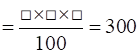According to this the simple interest for 4, 5, 6 years will be 400,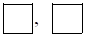respectively.

From this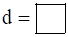and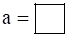Amount of simple interest after 20 years

tn + a + (n – 1) d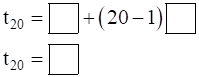Amount of simple interest after 20 years is =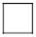Given: Principal Amount P = 1000

Rate of interest R = 10%

Also,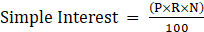Simple interest after 1 year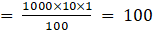Simple interest after 2 year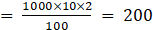Simple interest after 3 year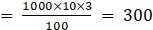According to this the simple interest for 4, 5, 6 years will be 400,

500, 600 respectively.

Let first term a = 100

Second term t1 = 200

Third term t3 = 300

Common difference d = t3 – t2 = 300 – 200 = 100

Amount of simple interest after 20 years

We use nth term of an A.P. formula

tn = a + (n – 1)d

where n = no. of terms

a = first term

d = common difference

tn = nth terms

⇒ t20 = 100 + (20 – 1) × 100

⇒ t20 = 100 + 19 × 100

⇒ t20 = 100 + 1900 = 2000

## PDF FILE TO YOUR EMAIL IMMEDIATELY PURCHASE NOTES & PAPER SOLUTION. @ Rs. 50/- each (GST extra)

SUBJECTS

HINDI ENTIRE PAPER SOLUTION

MARATHI PAPER SOLUTION
SSC MATHS I PAPER SOLUTION
SSC MATHS II PAPER SOLUTION
SSC SCIENCE I PAPER SOLUTION
SSC SCIENCE II PAPER SOLUTION
SSC ENGLISH PAPER SOLUTION
SSC & HSC ENGLISH WRITING SKILL
HSC ACCOUNTS NOTES
HSC OCM NOTES
HSC ECONOMICS NOTES
HSC SECRETARIAL PRACTICE NOTES

2019 Board Paper Solution

HSC ENGLISH SET A 2019 21st February, 2019

HSC ENGLISH SET B 2019 21st February, 2019

HSC ENGLISH SET C 2019 21st February, 2019

HSC ENGLISH SET D 2019 21st February, 2019

SECRETARIAL PRACTICE (S.P) 2019 25th February, 2019

HSC XII PHYSICS 2019 25th February, 2019

CHEMISTRY XII HSC SOLUTION 27th, February, 2019

OCM PAPER SOLUTION 2019 27th, February, 2019

HSC MATHS PAPER SOLUTION COMMERCE, 2nd March, 2019

HSC MATHS PAPER SOLUTION SCIENCE 2nd, March, 2019

SSC ENGLISH STD 10 5TH MARCH, 2019.

HSC XII ACCOUNTS 2019 6th March, 2019

HSC XII BIOLOGY 2019 6TH March, 2019

HSC XII ECONOMICS 9Th March 2019

SSC Maths I March 2019 Solution 10th Standard11th, March, 2019

SSC MATHS II MARCH 2019 SOLUTION 10TH STD.13th March, 2019

SSC SCIENCE I MARCH 2019 SOLUTION 10TH STD. 15th March, 2019.

SSC SCIENCE II MARCH 2019 SOLUTION 10TH STD. 18th March, 2019.

SSC SOCIAL SCIENCE I MARCH 2019 SOLUTION20th March, 2019

SSC SOCIAL SCIENCE II MARCH 2019 SOLUTION, 22nd March, 2019

XII CBSE - BOARD - MARCH - 2019 ENGLISH - QP + SOLUTIONS, 2nd March, 2019

HSC Maharashtra Board Papers 2020

(Std 12th English Medium)

HSC ECONOMICS MARCH 2020

HSC OCM MARCH 2020

HSC ACCOUNTS MARCH 2020

HSC S.P. MARCH 2020

HSC ENGLISH MARCH 2020

HSC HINDI MARCH 2020

HSC MARATHI MARCH 2020

HSC MATHS MARCH 2020

SSC Maharashtra Board Papers 2020

(Std 10th English Medium)

English MARCH 2020

HindI MARCH 2020

Hindi (Composite) MARCH 2020

Marathi MARCH 2020

Mathematics (Paper 1) MARCH 2020

Mathematics (Paper 2) MARCH 2020

Sanskrit MARCH 2020

Important-formula

THANKS